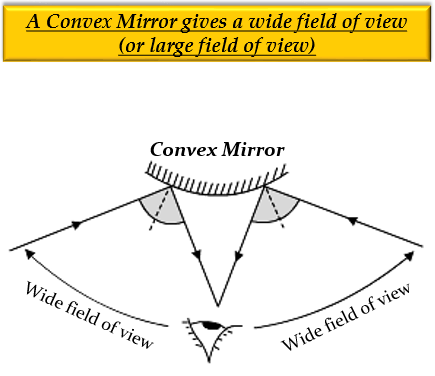# Linear magnification (m) produced by a rear view mirror fitted in vehicles:(a) is equal to one (b) is less than one(c) is more than one (d) can be more less than one depending on the position of object

(b) is less than one

Explanation

Linear magnification $(m)$ produced by a rear view mirror, installed in vehicles, is less than one $(m And, a convex mirror is a mirror that always forms images which are smaller than the object, so its linear magnification$(m)\$ is always less than 1.

In vehicles, a convex mirror is fitted as a rear view mirror because it gives a wider field of view, and always forms virtual, erect, and small-sized images of a large number of objects at the same time.Updated on: 10-Oct-2022

34 Views